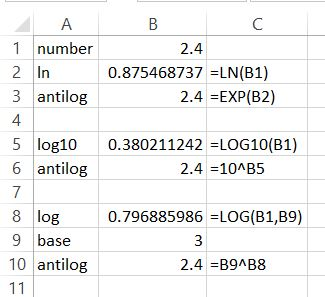The antilog, also known as the inverse logarithm, is a mathematical operation that can be used to reverse the effect of a logarithm. In Excel, the antilog can be calculated using the EXP function, which returns the exponential value of a given number. In this Excel tutorial, you will learn how to handle antilog in Excel.

## What is antilog?

The exponential function, denoted by e^x, is the inverse of the natural logarithm function. The antilog of a number is simply the exponential value of the logarithm of that number. In other words, if y = log(x), then x = e^y.

Sometimes, showing is better than describing. Here is the equation which will show you what is antilog.

antilogxY = Xy

I hope you understand now.

There are different types of logarithms in Excel. To calculate antilog, you need to calculate the opposite of the logarithm calculations.

### Natural logarithm

ln(x)=y

#### Antilog of natural logarithm

=exp(y)=x

Antilog ln formula is =EXP(B2) in my example.

So to calculate the antilog of a natural logarithm, you need to calculate the power of the number.

It’s important to note that the EXP function in Excel uses a natural logarithm base, which is denoted by e. This means that the antilog of a number in Excel is calculated using the exponential function with base e.

In some cases, you may need to use a different logarithm base, such as base 10.

### Log base 10

=log10(x)=y

#### Antilog of log 10

=10^y=x

Antilog log10 formula is =10^B5 in my example.

To calculate the inverse of log base 10 you need to power 10 to the base of the result of log10.

### Logarithm

=log(x,base)=y

=base^y=x

Antilog log formula is =B9^B5 in my example.

To get the antilog of a logarithm, you need to power base the result.As you can see, in every example, calculations work because antilog is equal to the number.

## Practical Applications of Antilogs in Excel

### Compound Interest Calculation

One of the most common applications of antilogs is in compound interest calculations. When you know the interest rate, principal amount, and time period, you can calculate the final amount using the antilogarithmic function.

For example, suppose you have \$1,000 as the principal amount, an annual interest rate of 5%, and you want to calculate the amount after 5 years:

Final Amount (A) = Principal Amount (P) * e^(Annual Interest Rate (r) * Time Period (t))

## Growth and Decay Modeling

Antilogs are also useful for modeling exponential growth or decay scenarios. For instance, in population growth, the compound interest of investments, or the decay of radioactive elements, antilogs can help predict future values based on the rate of change.

## Scientific and Engineering Calculations

In scientific and engineering calculations, antilogs are frequently used to reverse the effect of logarithmic measurements. For example, in chemistry, the pH scale uses logarithmic values to measure acidity. You can use antilogs to convert pH values back to hydrogen ion concentration.